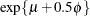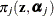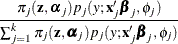OUTPUT Statement

OUTPUT <OUT=SAS-data-set>
<keyword<(keyword-options)> <=name>> …
<keyword<(keyword-options)> <=name>> </ options> ;

The OUTPUT statement creates a data set that contains observationwise statistics that are computed after fitting the model. By default, all variables in the original data set are included in the output data set. You can use the ID statement to limit the variables copied from the input data set to the output data set.

The output statistics are computed based on the parameter estimates of the converged model if the parameters are estimated by maximum likelihood. If a Bayesian analysis is performed, the output statistics are computed based on the arithmetic mean in the posterior sample. You can change to the maximum posterior estimate with the ESTIMATE=MAP option in the BAYES statement.

You can specify the following syntax elements in the OUTPUT statement before the slash (/).

OUT=SAS-data-set

specifies the name of the output data set. If the OUT= option is omitted, the procedure uses the DATAn convention to name the output data set.

keyword<(keyword-options)> <=name>

specifies a statistic to include in the output data set and optionally assigns the variable the name name. If you do not provide a name, the FMM procedure assigns a default name based on the type of statistic requested. If you provide a name for a statistic that leads to multiple output statistics, the name is modified to index the associated component number.

You can use the keyword-options to control which type of a particular statistic is computed. The following are valid values for keyword and keyword-options:

PREDICTED<(COMPONENT | OVERALL)>
PRED<(COMPONENT | OVERALL)>
MEAN<(COMPONENT | OVERALL)>

requests predicted values (predicted means) for the response variable. The predictions in the output data set are mapped onto the data scale in all cases except for a binomial or binary response with events/trials syntax and when PREDTYPE=COUNT has not been specified. In that case the predictions are predicted success probabilities.

The default is to compute the predicted value for the mixture (OVERALL). You can request predictions for the means of the component distributions by adding the COMPONENT suboption in parentheses. The predicted values for some distributions are not identical to the parameter modeled as. For example, in the lognormal distribution the predicted mean iswhereandare the parameters of an underlying normal process; see the section Log-Likelihood Functions for Response Distributions for details.

RESIDUAL<(COMPONENT | OVERALL)>
RESID<(COMPONENT | OVERALL)>

requests residuals for the response or residuals in the component distributions. Only raw residuals on the data scale are computed (observed minus predicted).

VARIANCE<(COMPONENT | OVERALL)>
VAR<(COMPONENT | OVERALL)>

requests variances for the mixture or the component distributions.

LOGLIKE<(COMPONENT | OVERALL)>
LOGL<(COMPONENT | OVERALL)>

requests values of the log-likelihood function for the mixture or the components. For observations used in the analysis, the overall computed value is the observations’ contribution to the log likelihood; if a FREQ statement is present, the frequency is accounted for in the computed value. In other words, if all observations in the input data set have been used in the analysis, adding the value of the log-likelihood contributions in the OUTPUT data set produces the negative of the final objective function value in the Iteration History table. By default, the log-likelihood contribution to the mixture is computed. You can request the individual mixture component contributions with the COMPONENT suboption.

MIXPROBS<(COMPONENT | MAX)>
MIXPROB<(COMPONENT | MAX)>
PRIOR<(COMPONENT | MAX)>
MIXWEIGHTS<(COMPONENT | MAX)>

requests that the prior weightsbe added to the OUTPUT data set. By default, the probabilities are output for all components. You can limit the output to a single statistic, the largest mixing probability, with the MAX suboption.

Note: The keyword prior is used here because of long-standing practice to refer to the mixing probabilities as prior weights. This must not be confused with the prior distribution and its parameters in a Bayesian analysis.

POSTERIOR<(COMPONENT | MAX)>
POST<(COMPONENT | MAX)>
PROB<(COMPONENT | MAX)>

requests that the posterior weightsbe added to the OUTPUT data set. By default, the probabilities are output for all components. You can limit the output to a single statistic, the largest posterior probability, with the MAX suboption.

Note: The keyword posterior is used here because of long-standing practice to refer to these probabilities as posterior probabilities. This must not be confused with the posterior distribution in a Bayesian analysis.

LINP
XBETA

requests that the linear predictors for the models be added to the OUTPUT data set.

CLASS | CATEGORY | GROUP

adds the estimated component membership to the OUTPUT data set. An observation is associated with the component that has the highest posterior probability.

MAXPOST | MAXPROB

adds the highest posterior probability to the OUTPUT data set.

A keyword can appear multiple times. For example, the following OUTPUT statement requests predicted values for the mixture in addition to the predicted means in the individual components:

 output out=fmmout pred=MixtureMean pred(component)=CompMean;


In a three-component model, this produces four variables in the fmmout data set: MixtureMean, CompMean_1, CompMean_2, and CompMean_3.

You can specify the following options in the OUTPUT statement after a slash (/).

ALLSTATS

requests that all statistics are computed. If you do not use a keyword to assign a name, the FMM procedure uses the default name.

NOVAR

requests that variables from the input data set not be added to the output data set. This option does not apply to variables listed in the BY statement or to variables listed in the ID statement.

PREDTYPE=PROB | COUNT

specifies the type of predicted values that are produced for a binomial or binary response with events/trials syntax. If PREDTYPE=PROB, the predicted values are success probabilities. If PREDTYPE=COUNT, the predicted values are success counts. The default is PREDTYPE=PROB.